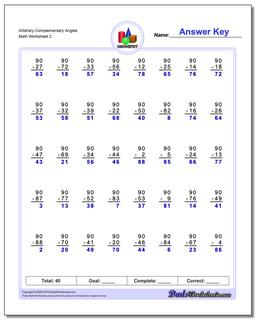# Math Worksheets: Basic Geometry: Basic Geometry: Arbitrary Complementary Angles (Second Worksheet)## Arbitrary Complementary Angles (Second Worksheet)

PropertyValue
DescriptionArbitrary Complementary Angles: Geometry worksheets with subtraction problems for finding complementary angles (Angles whose sum is 90 degrees). (Second Worksheet)
Resource TypeWorksheet